For your grading system, you can choose Total points or Weighted by category grading. In both, grades are calculated for you, and you can let students see their overall grade.

If you don’t want to use a grading system, you can choose No overall grade. Grades won’t be calculated and students can’t see an overall grade.

You can also organize classwork with grade categories, such as Essays, Homework, and Tests. For example, if your class has 4 essay assignments, you can organize them in an Essays category.

Adding grading systems and creating grade categories is only available in the web version of Classroom.

To have grades calculated for you and an option to let students see their overall grade, use Total points or Weighted by category grading.

An overall grade is calculated for you by dividing the total points a student earns by the total points possible in the class. You can create grade categories and set point values for classwork in each category. For example:
 Essay category Test category Student points/Points possible Overall grade Essay 1: 90/100 Test 1: 95/100 185/200 92.5%
The table shows that a student scored 90 points out of 100 on an essay, and 95 points out of 100 on a test. The student earned a total of 185 points out of 200 possible in the class. Divided, this gives an overall grade of 92.5%.

Weighted by category grading

You assign weights (percentages) to grade categories, and an overall grade is calculated for you. This system calculates as follows:
1. Finds a category’s average.
2. Multiplies each category’s average by its weight (as a decimal).
3. Adds the category scores for an overall grade out of 100%.
 Essay category:  50% of grade Test category: 50% of grade Overall grade out of 100% Essay 1: 70/100 Test 1: 90/100 Essay 2: 80/100 Test 2: 100/100 (70 + 80) ÷ 2 = 75 avg.  75 x .5 = 37.5 (90 + 100) ÷ 2 = 95 avg. 95 x .5 = 47.5 Category score = 37.5% Category score = 47.5% Overall grade: 37.5 + 47.5 = 85%

The table shows weighted by category grading. In the Essay category, the student averaged 75. Multiplied by the category weight, .5, this gives 37.5% for Essays. In the Test category, the student averaged 95. Multiplied by the category weight, .5, this gives 47.5% for Tests. Adding the category scores (37.5 + 47.5) gives the student an overall grade of 85%.

Set up a grading system

After you select a grading system, you can add grade categories. Grade categories are required with Weighted by category grading, but can also be used with Total points grading or No overall grade.

Select a grading system

You can only select a grading system in the web version of Classroom.

2. Click your class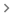Settings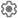.3. Next to Overall grade calculation, select one:
• No overall grade—Grades aren’t calculated for students. Students can’t see an overall grade.
• Total points—Divides total points earned by total points possible. You can let students see an overall grade.
• Weighted by category—Adds the scores across categories. You can let students see an overall grade.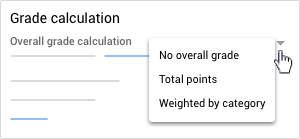4. (Optional) To make the overall grade visible to students on their profile page, click Show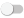.
Note: This option isn’t available when grade calculation is set to No overall grade.5. In the top-right corner, click Save.

Create grade categories for No overall grade or Total points grading

For these options, you assign default point values to the grade categories you create.

Note: You can only create grade categories in the web version of Classroom.

2. Click your classSettings.3. Next to Overall grade calculation, select No overall grade or Total points from the menu.5. Enter a grade category.
6. Under Default points, enter a whole number.7. (Optional) To add another grade category, repeat steps 4–6.
8. In the top-right corner, click Save.

Create grade categories for Weighted by category grading

For this option, you assign percentages to the categories you create.

Note: You can only create grade categories in the web version of Classroom.

2. Click your classSettings.3. Next to Overall grade calculation, select Weighted by category from the menu.5. Enter a grade category
6. Under Percentage, enter a whole number.7. (Optional) To add another grade category, repeat steps 4–6.
8. In the top-right corner, click Save.
Note: To save your settings, the categories must add up to 100%.

Remove a grade category

You can remove grade categories. Removing a grade category:

• Removes the grade category from the corresponding classwork.
• Doesn't delete the classwork itself.
2. Click your classSettings.3. Next to the grade category you want to remove, click Remove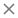.